• Introduction
• What is in this manual
• What is Caspoc
• User interface
• Introduction
• Starting
• Simulation
• Editing
• Viewing and printing
• Getting Started
• Basic editing
• Simulation in the time domain
• Basic User Interface Topics
• Editing
• Simulation
• Viewing
• Library
• Reports
• Project management
• Circuit and Block Diagram Components
• Introduction
• Cscript and user defined functions
• Component parameters
• Modeling Topics
• Introduction
• Power Electronics
• Semiconductors
• Electrical Machines
• Electrical drives
• Power Systems
• Mechanical Systems
• Thermal Systems
• Magnetic Circuits
• Green Energy
• Coupling to FEM
• Experimenter
• Analog hardware description language
• Embedded C code Export
• Coupling to Spice
• Small Signal Analysis
• Matlab coupling
• Tips and tricks
• Appendices

## Open a scope.

If the simulation is finished, the results are stored in the scopes. You can open a sope by clicking it with the right mouse button: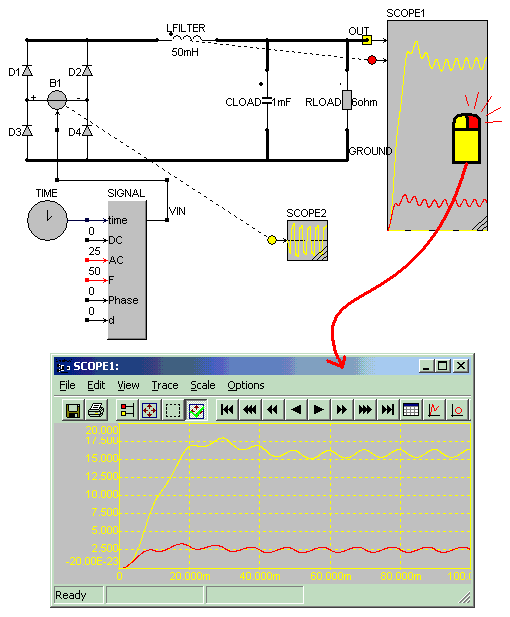The scope window will open and the simulation results will be displayed as function of time. You can go through the simulation results by pressing the arrow and PgUp/PgDn/Home/End keys on your keyboard: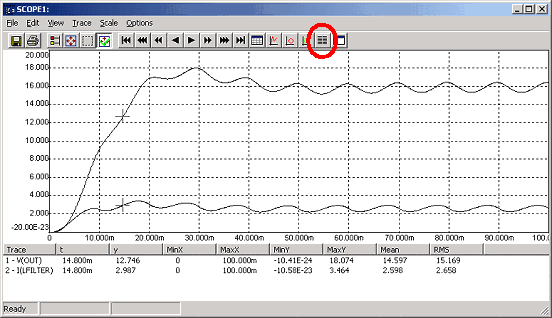To see the second input as function of the first input, for example the load curve of an induction machine during start up, select View/XY Writer from the scope menu. For the rectifier sample, the inductor current as function ofthe output voltage of the rectifier is shown below: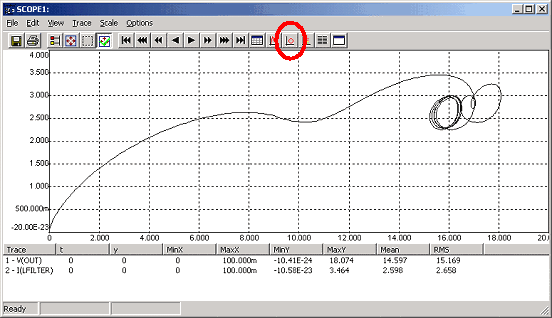To see the harmonics of the input current, the simulation has to be continued until the steady state is reached and the input current through the source B is periodic.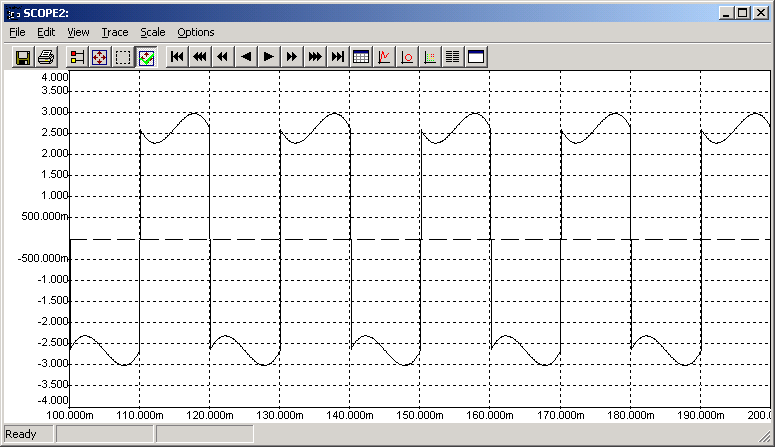The number of harmonics is set in the DFT parameters dialog box that is selected at Scale/DFT Parameters from the scope menu.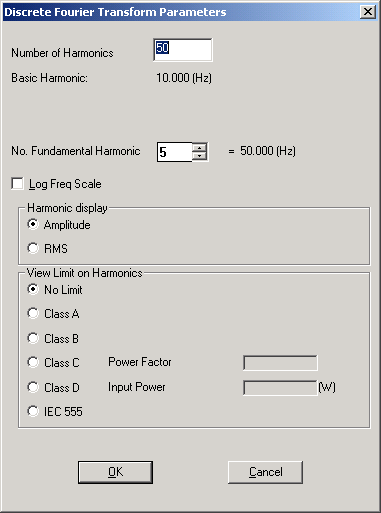To see the harmonics select View/DFT from the scope window.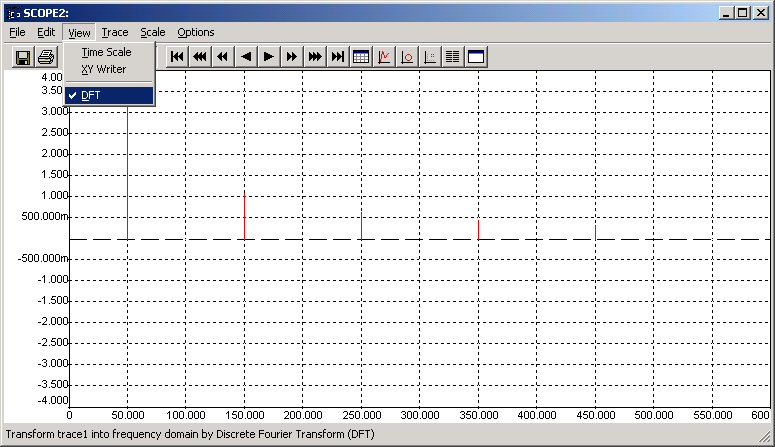The above scope shows the 1,3,5,7 and 9th harmonic. Since only harmonics up to 500Hz are calculated, the 11th harmonic is not shown any more. Using the arrow keys, you can see the numerical values of the harmonics.

When the numerical results are displayed, a number of factors are calculated and shown in the listbox. These factors are calculated as:

Xminmin(x1..xn)
Xmaxmax(x1..xn)
Yminmin(y1..yn)
Ymaxmax(y1..yn)
Mean value
 N (1/N) Σ yi i=0
RMS value
 N SQRT { (1/N) Σ yi2 } i=0
Peak-to-peak valuemax(y1..yn)-min(y1..yn)
Mean absolute value
 N (1/N) Σ |yi| i=0
AC RMS valueSQRT{ y2RMS- y2Mean }
Integral
 N Σ yi*Δ x i=0
RippleSQRT{ y2RMS/y2Mean -1 }
Ripple factor(ymax-ymin)/|yMean|
Harmonic contentSQRT{1- ( 1/y2Ripple)}
Form factoryRMS/yMean
Crest factormax(|ymax|,|ymin|)/yRMS